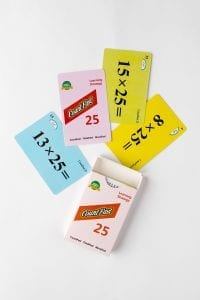# CountFast 25 Home Activities

####OVERVIEW & PURPOSE

Our final week of the 3rd Grade CountFast program focuses on specific mental strategies for multiplying with the numbers 25 and 75. Spend 15 minutes each day on one of the activities listed in this module.  Card decks should go home with students each day for additional practice with a parent at home. Each week, a new deck is introduced, and the previous deck is for the student to keep at home for continued practice.

#### EDUCATION STANDARDS

1.    CCSS.Math.Content.3.OA.B.5
Apply properties of operations as strategies to multiply and divide.
2.  NCTM Standard: develop a sense of whole numbers and represent and use them in flexible ways, including relating, composing, and decomposing numbers

#### OBJECTIVES

1. Quickly, mentally multiply by 25 and 75.
2. Develop fluency with multiplication calculations.
DAYS 1 and 2:Use the yellow cards from the deck.  The mental strategy lesson learned today is how to multiply any number by 25.  We can think of 25 as being one-fourth of 100.  So it makes sense that we could multiply the original number by 100, and then divide the answer by 4.  Practice this strategy with your child and encourage him/her to calculate the answers faster each round, keeping track of his/her time on the time record card.  Your child will be excited every time he/she can get through the cards faster than the time before!

• 8 x 25 = 800 ÷ 4                                         1) 15 x 25 = 1500 ÷ 4               1) 20 x 25 = 2000 ÷ 4
• 800 ÷ 4= 200                                              2) 1500 ÷ 4 = 375                     2) 2000 ÷ 4 = 500

(same as 8 ÷ 4, add back zeros)

DAYS 3 and 4:

Use the same procedure as Days 1 and 2.  This time, your child will use the pink cards in the deck to multiply any number by 75.  We learned in class that we can think of 75 as being the same thing as 3 X 25.  To multiply by 75, first multiply the original number by 3.  Then take that answer and multiply it by 100.  Finally, divide that answer by 4 (you can cut it in half twice instead of dividing by 4). We will practice this strategy for two days.  Help you child keep track of how long it takes to use the strategy to answer all of the blue cards.  Celebrate each time your child records a faster time.

For example: 13 x 75

First, we multiply the number by 3:            13 X 3 = 39

Now, we multiply by 100:          39 X 100 = 3900

The last step is to divide by 4, and in order to do so we just divide twice by 2:

3900 / 2 = 1950                                          1950 / 2 = 975

We end up with, 13 x 75 = 975

DAY 5:

Review the strategies for multiplying by 25 and 75 using the entire deck of cards.

**Please keep all of the decks of cards and continue to use with your child throughout the year.  Thank you for participating in the CountFast program each evening at home!  Together, we are creating children with strong mental computation skills! **

Stay SharpFree flash card decks to keep kids entertained and sharp!

To help you keep your kids math sharp during this crisis, we're giving you 3 free CountFast flash card decks for just \$6 in shipping.  Just have them take the quick quiz for their grade at the link below and you'll get a discount code to use on any three decks.